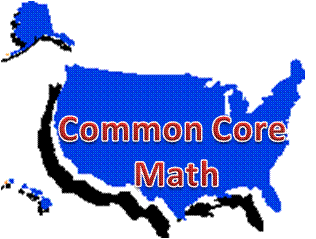# Common Core High School Number and Quantity Teaching and Learning Resources

## Domain: Q: Quantities• Q-A: Reason quantitatively and use units to solve problems### Q-A: Reason quantitatively and use units to solve problems

Standards:

• Q-A.1. Use units as a way to understand problems and to guide the solution of multi-step problems; choose and interpret units consistently in formulas; choose and interpret the scale and the origin in graphs and data display.
• Q-A.2. Define appropriate quantities for the purpose of descriptive modeling.
• Q-A.3. Choose a level of accuracy appropriate to limitations on measurement when reporting quantities.

#### What is Measurement?Technology-enhanced investigations:

LearnZillion:

National Institute of Standards and Technology, an agency of the US Dept. of Commerce, includes resources to learn the metric system.  The following are among those:

Ohio Resource Center Tutorials for High School Mathematics YouTube videos:

Shodor Interactivate: Triangle Explorer: Learners study the nature of triangles and their area, and can investigate problem solving strategies.

Wolfram Demonstrations Project: Download the free Wolfram CDF player to interact with the following manipulative.  There  is 1 manipulative addressing Q-A.2:

Multiple Choice:

Khan Academy: Practice questions with videos.

Constructed-response:

Consortium for Mathematics and Its Applications (COMAP): Mathematical Modeling Handbook (Sample).  This sample  mathematical modeling module aligns to Common Core Standards.  It contains teacher guidance for implementation and student questions to complete during their investigation:

• The module For the Birds aligns to number and quantity standards Q-A.1 and Q-A.2, and algebra standard CED-A.4.

Khan Academy: Working with Units unit.  Practice questions with videos.

MathBitsNotebook: Algebra 1: Working with Units includes lessons on significant figures and accuracy and error in measurement, then practice problems on working with units and error in measurement.  Use with Q-A.1, Q-A.2, and Q-A.3.

ScienceSpot.net: Metric Mania includes lessons on length, mass, volume, density and constructed response conversion practice worksheets with answers.

Illustrative Mathematics: Number and Quantity:

Inside Mathematics: MARS Task: Swimming Pool: This task aligns with standards Q-A.1, Q-A.2, and Q-A.3.  Students work with trapezoids, rates and time graphs in a real context.

Mathematics Assessment Project: Standards: High School: Number and Quantity: Quantities:

Mathematics Vision Project, Secondary 1 Student Edition: Getting Ready Module: This module contains 10 classroom tasks.  Task 3: Serving Up Symbols; and Task 4: Examining Units align with Q-A.1.  Task 1: Checkerboard Borders; and Task 2: Building More Checkerboard Borders align with Q-A.2.  Tasks 1-3 also include algebra standard SSE-A.1.  The remaining six tasks addressed in this module include algebra standards REI-A.1, REI-B.3, and CED-A.4.

NCTM's Reasoning and Sense Making Task Library: Each includes the task overview, teacher notes for its use in the classroom, and student activity sheet.

• Fuel for Thought: Aligns with Q-A.1, Q-A.2, and mathematical practice standards 2, 3, and 4.
• Cash or Gas?: Aligns with Q-A.1, Q-A.2, statistics standard MD-B.5.b, and mathematical practice standards 1, 3, 4, and 5.